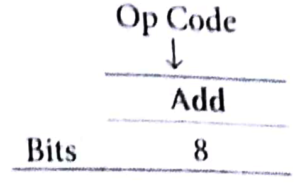# Machine Instructions in Computer Organization Study Notes with Examples

## Machine Instructions

### Computer Instruction

A binary code used for specifying micro operations for computer. Instruction Code Group of bits used to instruct the CPU to perform an specific operation.

Instruction Set: Collection of instructions.

Instruction Representation: Each instruction has a unique bit pattern, but for human beings a corresponding symbolic representation has been defined. e.g., ADD, SUB, LOAD, etc.

### Instruction Cycles

#### Instruction cycle consists of following phases

• Fetching an instruction from memory.
• Encoding the instruction.
• Execution of the instruction.

### Instruction Format

An instruction consists of bits and these bits are grouped up to make fields. Some fields in instruction format are as follows

1. Opcode which tells about the operation to be performed.
3. Mode field specifying the way the operand or effective address is

### Different types of Instruction formats

#### some common types are as given below

• This system contains three address fields (address of operand 1, address of operand 2 and address where result needs to be put).
• The address of next instruction is held in a CPU register called Program Counter (PC).

Here, the number of bytes required to encode an instruction is 10 bytes i.e., each address requires 24 bit = 3 bytes. Since, there are three addresses and one opcode field so,

3 x 3 +1=10 bytes.

The number of memory access required is 7 words, i.e., 4 words for instruction fetch, 2 words for operand fetch and 1 word for result to be placed back in memory.

• In this format, two addresses and an operation field is there.
• The result is stored in either of the operand address e., either in address of first operand or in the address of second operand.
• CPU register called Program Counter (PC) contains the address of next

• One address field and an operation field.
• This address is of the first operand.
• The second operand and the result are stored in a CPU register called Accumulator Register (AR). Since, a machine has only one accumulator, it needs not be explicitly mentioned in the instruction.
• A CPU register (i.e., Program Counter (PC) holds the address of next
• In this scenario, two extra instructions are required to load and store the accumulator contents.

• Number of bits required to encode an instruction is 4 bytes. i.e., each address requires 24 bits = 3 bytes. Since, there are one address and one operation code field, 1* 3 + 1= 4 bytes.
• The number of memory access required is 3 words i.e., 2 words for instruction fetch +1 word for code for operand fetch.

### Key Points

• Total number of bytes to encode an instruction = number of address fields * Bytes required to store an address + bytes required to store operation code. 2 * 3 + 1 = 7 bytes.
• The number of memory access required is 6 words e., 3 words for instruction fetch +2 words for operand fetch +1 word for result to be placed back in memory.

• Here, an stack is included in the CPU for performing arithmetic and logic instructions with no addresses.
• The operands are pushed onto the stack from memory and ALU operations are implicitly performed on the top elements of the stack.
• The address of the next instruction is hold in a CPU register called program